# Guerry data: Multivariate Analysis

#### 2023-05-05

André-Michel Guerry’s Essai sur la Statistique Morale de la France collected data on crimes, suicide, literacy and other “moral statistics” for various départements in France. He provided the first real social data analysis, using graphics and maps to summarize this multivariate dataset. One of his main goals in this ground-breaking study was to determine if the prevalence of crime in France could be explained by other social variables.

In 1833, the scatterplot had not yet been invented; the idea of a correlation or a regression was still 50 years in the future . Guerry displayed his data in shaded choropleth maps and semi-graphic tables and argued how these could be seen as implying systematic, lawful relations among moral variables.

In this analysis, we ignore the spatial context of the départements and focus on multivariate analyses of the the data set.

We will primarily use the following packages, so load them now.

library(Guerry)         # Guerry data
library(car)            # better scatterplots
library(effects)        # Effect Displays for Linear Models
library(ggplot2)        # Elegant Data Visualisations Using the Grammar of Graphics
library(ggrepel)        # better handling of text labels
library(patchwork)      # combine plots
library(heplots)        # Hypothesis-Error plots
library(candisc)        # Visualizing Generalized Canonical Discriminant Analysis
library(dplyr)          # A Grammar of Data Manipulation
library(tidyr)          # Tidy Messy Data
data(Guerry)

## Guerry data set

Guerry’s (1833) data consisted of six main moral variables shown in the table below. He wanted all of these to be recorded on aligned scales so that larger numbers consistently reflected “morally better”. Thus, four of the variables are recorded in the inverse form, as “Population per …”.

Name Description
Crime_pers Population per crime against persons
Crime_prop Population per crime against property
Literacy Percent of military conscripts who can read and write
Donations Donations to the poor
Infants Population per illegitimate birth
Suicides Population per suicide

The Guerry data set also contains:

• dept and Department, the French ID numbers and names for the 86 départements of metropolitan France in 1830, including Corsica.
• Region: a factor with main levels “N”, “S”, “E”, “W”, “C”. Corsica is coded as NA.
• A collection of 14 other related variables from other sources at the same time. See ?Guerry for their precise definitions.
names(Guerry)[-(1:9)]
#>   "MainCity"        "Wealth"          "Commerce"        "Clergy"
#>   "Crime_parents"   "Infanticide"     "Donation_clergy" "Lottery"
#>   "Desertion"       "Instruction"     "Prostitutes"     "Distance"
#>  "Area"            "Pop1831"

Among these, as other aspects of criminal behavior, we see crime against parents, Infanticide and Prostitutes. Clergy and Donations_clergy are considered to be measures of moral rectitude, potentially counteracting crime.

## Guerry’s questions

The main questions that concerned Guerry were whether indicators of crime could be shown to be related to factors which might be considered to ameliorate crime. Among these, Guerry focused most on Literacy defined as the number of military conscripts who could do more than mark an “X” on their enrollment form. A related variable is Instruction, the rank recorded from Guerry’s map; as defined, it is inversely related to Literacy.

Other potential explanatory variables are:

Donations (a measure of donations to the poor),

Donation_clergy (a measure of donations to clergy)
Clergy (the rank of number of Catholic priests in active service, per population)

# Multivariate visualization methods

Visualization methods for multivariate data take an enormous variety of forms simply because more than two dimensions of data offer exponentially increasingly possibilities. It is useful to distinguish several broad categories:

• data plots : primarily plot the raw data, often with annotations to aid interpretation (regression lines and smooths, data ellipses, marginal distributions)

• model plots : primarily plot the results of a fitted model, considering that the fitted model may involve more variables than can be shown in a static 2D plot. Some examples are: Added variable plots, effect plots, coefficient plots, …

• diagnostic plots : indicating potential problems with the fitted model. These include residual plots, influence plots, plots for testing homogeneity of variance and so forth.

• dimension reduction plots : plot representations of the data into a space of fewer dimensions than the number of variables in the data set. Simple examples include principal components analysis (PCA) and the related biplots, and multidimensional scaling (MDS) methods.

# Data plots

Data plots portray the data in a space where the coordinate axes are the observed variables.

• 1D plots include histograms and density estimates
• 2D plots are most often scatterplots
• for higher dimensions, biplots are often the most useful

## Density plots

It is useful to examine the distributions of the variables and density plots are quite informative. I want to do this for each of the 6 main variables, so I’ll use this trick of tidy data analysis with ggplot2:

1. Reshape the data from wide to long. This gives guerry_long, where the different variables are in a column labeled variable and the values are in value.
data("Guerry", package="Guerry")
guerry_long <- Guerry |>
filter(!is.na(Region)) |>
select(dept:Suicides) |>
pivot_longer(cols = Crime_pers:Suicides,
names_to = "variable",
values_to = "value")
guerry_long
#> # A tibble: 510 × 5
#>     dept Region Department variable   value
#>    <int> <fct>  <fct>      <chr>      <int>
#>  1     1 E      Ain        Crime_pers 28870
#>  2     1 E      Ain        Crime_prop 15890
#>  3     1 E      Ain        Literacy      37
#>  4     1 E      Ain        Donations   5098
#>  5     1 E      Ain        Infants    33120
#>  6     1 E      Ain        Suicides   35039
#>  7     2 N      Aisne      Crime_pers 26226
#>  8     2 N      Aisne      Crime_prop  5521
#>  9     2 N      Aisne      Literacy      51
#> 10     2 N      Aisne      Donations   8901
#> # ℹ 500 more rows
1. Plot the density, but make a different subplot by facet_wrap(~ variable). These plots all have different scales for the X and Y (density) values, so it is important to use scales="FREE". Moreover, I’m primarily interested in the shape of these distributions, so I suppress the Y axis tick marks and labels.
ggplot(data = guerry_long,
aes(x=value, fill=TRUE)) +
geom_density(alpha=0.2) +
geom_rug() +
facet_wrap(~variable, scales="free") +
theme_bw(base_size = 14) +
theme(legend.position = "none",
axis.ticks.y=element_blank(),
axis.text.y=element_blank())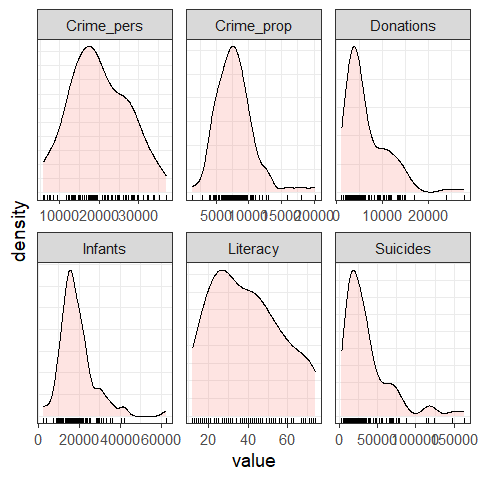You can see that all variables are positively skewed, Donations, Infants and Suicides particularly so, but not so much as to cause alarm.

It is also of interest to see whether and how these distributions differ according to Region. This is easy to do, using aes(... fill=Region)

col.region   <- colors()[c(149, 254, 468, 552, 26)] # colors for region
ggplot(data = guerry_long,
aes(x=value, fill=Region)) +
geom_density(alpha=0.2) +
geom_rug() +
facet_wrap(~variable, scales="free") +
scale_fill_manual(values=col.region) +
theme_bw(base_size = 14) +
theme(legend.position = "bottom",
axis.ticks.y=element_blank(),
axis.text.y=element_blank())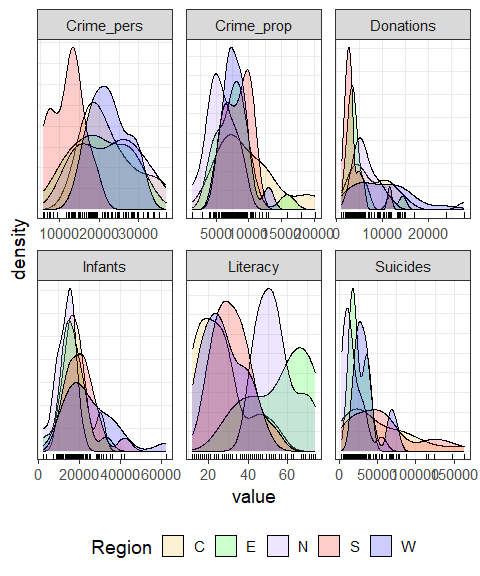For some variables, like Infants and Suicides the differences do not seem particularly large. However, both crime variables and Literacy show marked differences across region.

## Bivariate relations

Let’s start with plots of crime (Crime_pers and Crime_prop) in relation to Literacy. A simple scatterplot is not very informative. All that can be seen is that there is not much of a relation between personal crime and literacy.

ggplot(aes(x=Literacy, y=Crime_pers/1000), data=Guerry) +
geom_point(size=2)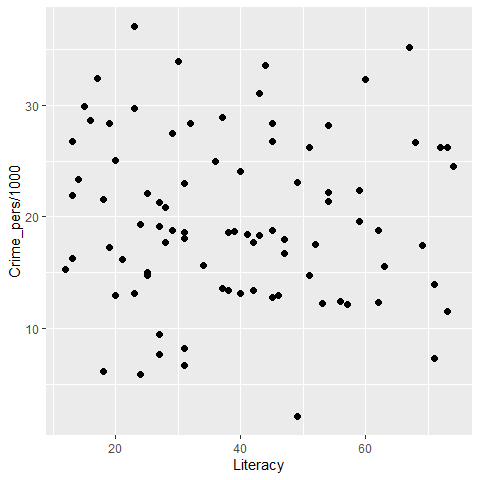More useful scatterplots are annotated with additional statistical summaries to aid interpretation:

• linear regression line,
• smoothed non-parametric (loess) curve, to diagnose potential non-linear relations,
• data ellipses, to highlight the overall trend and variability,
• point labels for potentially outlying or influential points.

I use ggplot2 here. It provides most of these features, except that to label unusual points, I calculate the Mahalanobis squared distance of all points from the grand means.

gdf <- Guerry[, c("Literacy", "Crime_pers", "Department")]
gdf$dsq <- mahalanobis(gdf[,1:2], colMeans(gdf[,1:2]), cov(gdf[,1:2])) ggplot(aes(x=Literacy, y=Crime_pers/1000, label=Department), data=gdf) + geom_point(size=2) + stat_ellipse(level=0.68, color="blue", size=1.2) + stat_ellipse(level=0.95, color="gray", size=1, linetype=2) + geom_smooth(method="lm", formula=y~x, fill="lightblue") + geom_smooth(method="loess", formula=y~x, color="red", se=FALSE) + geom_label_repel(data = gdf[gdf$dsq > 4.6,]) +
theme_bw()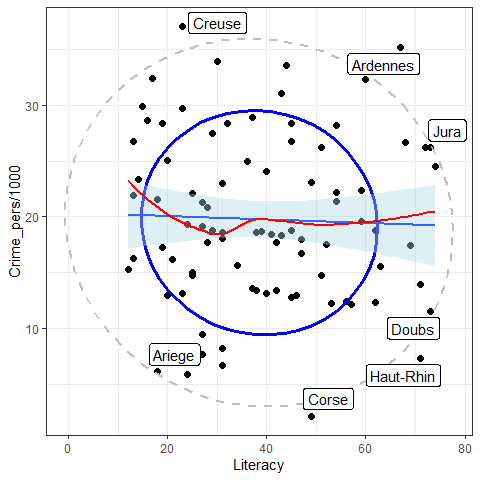The flat (blue) regression line and the nearly circular data ellipses show that the correlation is nearly zero; the smoothed (red) curve indicates that there is no tendency for a nonlinear relation.

Doing the same for crimes against property:

gdf <- Guerry[, c("Literacy", "Crime_prop", "Department")]
gdf$dsq <- mahalanobis(gdf[,1:2], colMeans(gdf[,1:2]), cov(gdf[,1:2])) ggplot(aes(x=Literacy, y=Crime_prop/1000, label=Department), data=gdf) + geom_point(size=2) + stat_ellipse(level=0.68, color="blue", size=1.2) + stat_ellipse(level=0.95, color="gray", size=1, linetype=2) + geom_smooth(method="lm", formula=y~x, fill="lightblue") + geom_smooth(method="loess", formula=y~x, color="red", se=FALSE) + geom_label_repel(data = gdf[gdf$dsq > 4.6,]) +
theme_bw()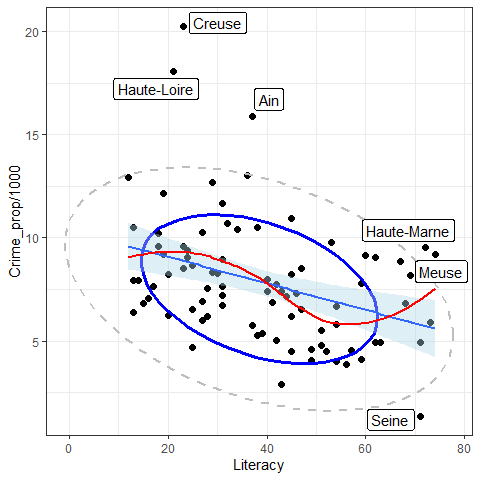So, somewhat surprisingly, increased literacy is associated with an increase in property crime (greater population per crime) as opposed to the situation with personal crime, which seems unrelated to literacy. Creuse again stands out as an unusual point, one that is likely to be influential in regression models.

## Reconnaisance plots

Reconnaisance plots attempt to give a bird’s-eye overview of a multivariate data set. For example, to see the relations among more than two variables we could turn to a scatterplot matrix or some other display to show all pairwise bivariate relations.

For these, my preferred package is car with the scatterplotMatrix function. GGally works within the the ggplot2 framework, but doesn’t have the flexibility I’d like.

library(car)          # Companion to Applied Regression
scatterplotMatrix(Guerry[,4:9],
ellipse=list(levels=0.68),
smooth=FALSE)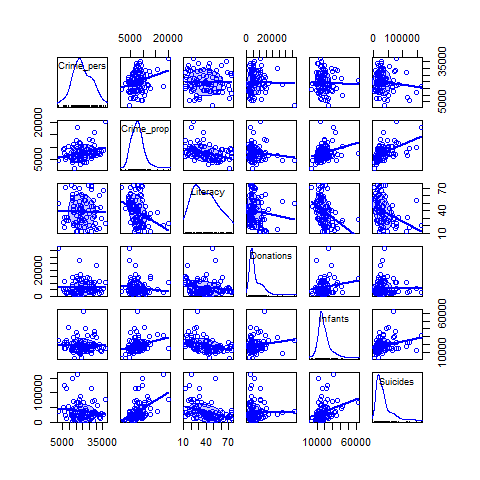### Corrgrams

Sometimes, particularly with more variables than this, we want to see a more schematic overview.
A correlation diagram or “corrgram” is a graphic display of a correlation matrix, allowing different renderings of the correlation between each pair of variables: as a shaded box, a pie symbol, a schematic data ellipse, and other options. This is implemented in the corrgram package . The panels in the upper and lower triangles can be rendered differently.

library(corrgram)             # Plot a Correlogram
corrgram(Guerry[,4:9], upper=panel.pie)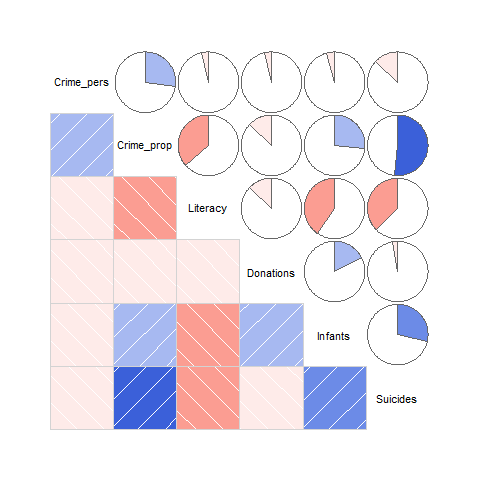Or, the data in each pairwise tile can be rendered with data ellipses and smoothed curves to show possible nonlinear relations.

Another feature is that the rows/column variables can be permuted to put similar variables together, using the order option, which arranges the variables according to similarity of their correlations.

corrgram(Guerry[,4:9],
upper=panel.ellipse,
order=TRUE,
lwd=2)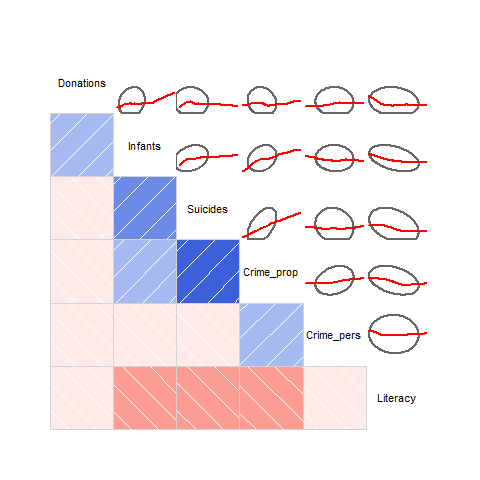Here, there are a number of pairwise plots that appear markedly nonlinear. For the main crime variables, the most nonlinear are that of personal crime vs. donations to the poor, and property crime vs. infants born out of wedlock and suicides. Literacy stands out here as having negative relations with all other variables.

An alternative analysis might include:

• converting the data to ranks.
• considering transformations of some of the variables

## Biplots

Rather than viewing the data in data space, a biplot shows the data in the reduced-rank PCA space that explains most of the variation of the observations. This is essentially a plot of the observation scores on the first principal component overlaid with vectors representing the variables projected into PCA space.

First, we use prcomp() to carry out the PCA. We’d like to visualize the result in relation to Region, so delete Corsica where Region is missing.

gdata <- Guerry[1:9]    # keep only main variables;
gdata <- Guerry[-86,]   # delete Corsica (Region==NA)

guerry.pca <- prcomp(gdata[,4:9], scale=TRUE)
print(guerry.pca, digits=3)
#> Standard deviations (1, .., p=6):
#>  1.463 1.096 1.050 0.817 0.741 0.584
#>
#> Rotation (n x k) = (6 x 6):
#>                PC1     PC2     PC3      PC4     PC5     PC6
#> Crime_pers -0.0659  0.5906 -0.6732  0.13973 -0.0102 -0.4172
#> Crime_prop -0.5123 -0.0884 -0.4765 -0.09861  0.1381  0.6884
#> Literacy    0.5118 -0.1294 -0.2090  0.00797  0.8213  0.0560
#> Donations  -0.1062  0.6990  0.4134 -0.47298  0.2742  0.1741
#> Infants    -0.4513  0.1033  0.3238  0.73031  0.3776 -0.0696
#> Suicides   -0.5063 -0.3569 -0.0169 -0.46220  0.2976 -0.5602

In the ggplot2 framework, biplots can be produced by the ggbiplot package, but this package is not on CRAN, so cannot be directly used here. Instead, the code below was run locally and the result included.

if(!require(ggbiplot)) remotes::install_github("vqv/ggbiplot")
library(ggbiplot) # A ggplot2 based biplot
ggbiplot(guerry.pca, groups=gdata$Region, ellipse=TRUE, var.scale = 3, varname.size = 5) + theme_bw() + labs(color="Region") + theme(legend.position = c(0.1, 0.8)) knitr::include_graphics("figures/ggbiplot.png")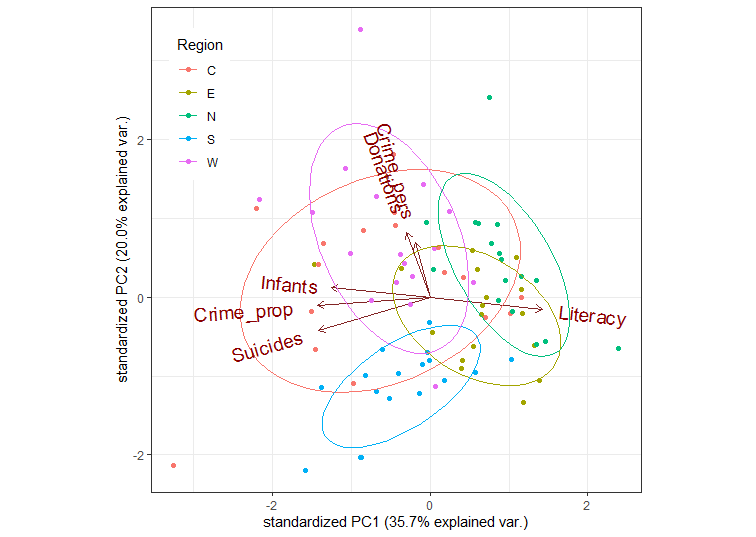This is OK, but there are many features of such plots that cannot be customized (line widths, colors, … ). I prefer those created using the heplots package. op <- par(mar=c(5,4,1,1)+.1) cols = colorspace::rainbow_hcl(5) covEllipses(guerry.pca$x,
group=gdata$Region, pooled=FALSE, fill=TRUE, fill.alpha=0.1, col=cols, label.pos=c(3,0,1,1,3), cex=2, xlim=c(-4,4), ylim=c(-4,4), xlab = "Dimension 1 (35.7 %)", ylab = "Dimension 2 (20.0 %)", cex.lab=1.4 ) points(guerry.pca$x, pch=(15:19)[Guerry$Region], col=cols[Guerry$Region])

candisc::vectors(guerry.pcarotation, scale=5, col="black", lwd=3, cex=1.4, pos = c(4,2,4,2,2,2), xpd=TRUE) abline(h=0, v=0, col=gray(.70))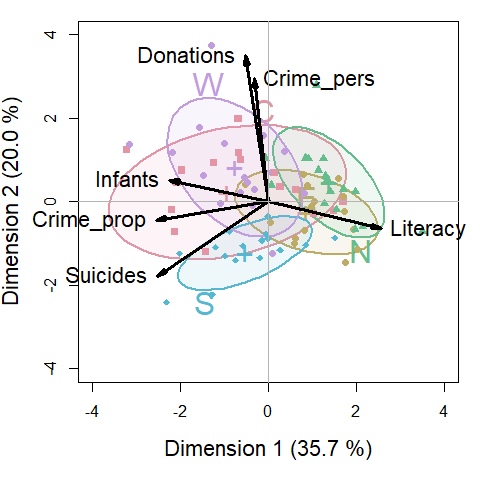An interpretation can be read from both the directions of the variable arrows and the relative positions of the ellipses representing the scatter of the component scores for the different regions. • The first component is largely aligned positively with Literacy and negatively with property crime, suicides and children born out of wedlock (Infants) • The second dimension reflects mainly the correlation of personal crime and donations to the poor. • The South region is generally lower on PC2, the West, generally higher. • The North stands out as being higher than the others on PC1; the West somewhat higher on PC2. # Models Here we illustrate: • Model based plots for linear regression models predicting personal crime and property crime • Multivariate analysis of variance (MANOVA) and HE plots for the joint relation of the crime variables to other predictors. ## Predicting crime: Univariate regression The simplest approach to predicting the crime variables would be to fit a separate multiple regression to each. crime.mod1 <- lm(Crime_pers ~ Region + Literacy + Donations + Infants + Suicides, data=Guerry) crime.mod2 <- lm(Crime_prop ~ Region + Literacy + Donations + Infants + Suicides, data=Guerry) Tests for the predictors are best obtained using car::Anova() which gives partial (Type II) tests, adjusting for other predictors, rather than the sequential (Type I) tests provided by stats::anova() Anova(crime.mod1) #> Anova Table (Type II tests) #> #> Response: Crime_pers #> Sum Sq Df F value Pr(>F) #> Region 1388267847 4 9.0398 5.005e-06 *** #> Literacy 77140249 1 2.0092 0.1604 #> Donations 54505520 1 1.4197 0.2372 #> Infants 102152 1 0.0027 0.9590 #> Suicides 205432 1 0.0054 0.9419 #> Residuals 2917886368 76 #> --- #> Signif. codes: 0 '***' 0.001 '**' 0.01 '*' 0.05 '.' 0.1 ' ' 1 Anova(crime.mod2) #> Anova Table (Type II tests) #> #> Response: Crime_prop #> Sum Sq Df F value Pr(>F) #> Region 52269436 4 2.0939 0.0898250 . #> Literacy 13366819 1 2.1419 0.1474514 #> Donations 9218353 1 1.4771 0.2279870 #> Infants 7577617 1 1.2142 0.2739759 #> Suicides 100890796 1 16.1665 0.0001355 *** #> Residuals 474296314 76 #> --- #> Signif. codes: 0 '***' 0.001 '**' 0.01 '*' 0.05 '.' 0.1 ' ' 1 These are somewhat disappointing if you look only at the significance stars: Only Region is significant for personal crime and only Suicides for property crime. There is no evidence for the argument, supported by the liberal hygenicists of Guerry’s time, that increased Literacy would reduce crime. For such models, we can understand the nature of the predicted effects using the effects package. The (marginal) effect for a given term gives the predicted values, averaging over all other terms in the model. plot(predictorEffects(crime.mod1, ~ Region + Literacy + Infants + Suicides), lwd=2, main="")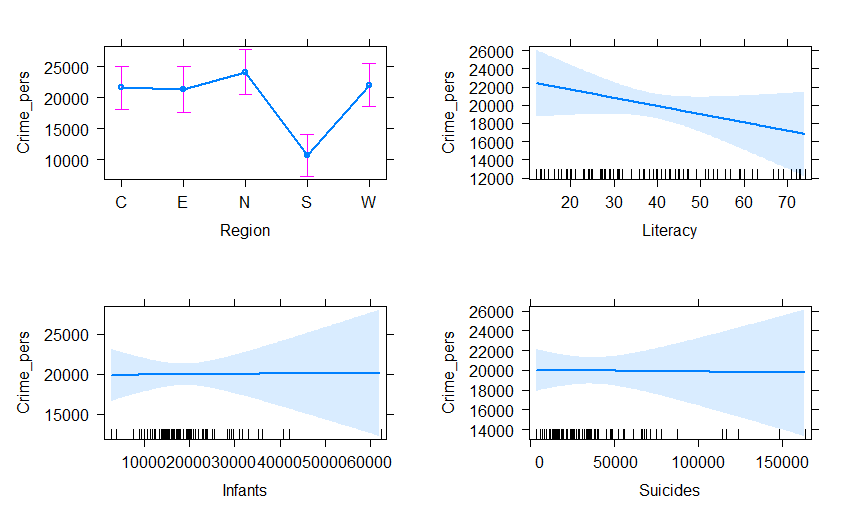Doing the same for property crime, we get: plot(predictorEffects(crime.mod2, ~ Region + Literacy + Infants + Suicides), lwd=2, main="")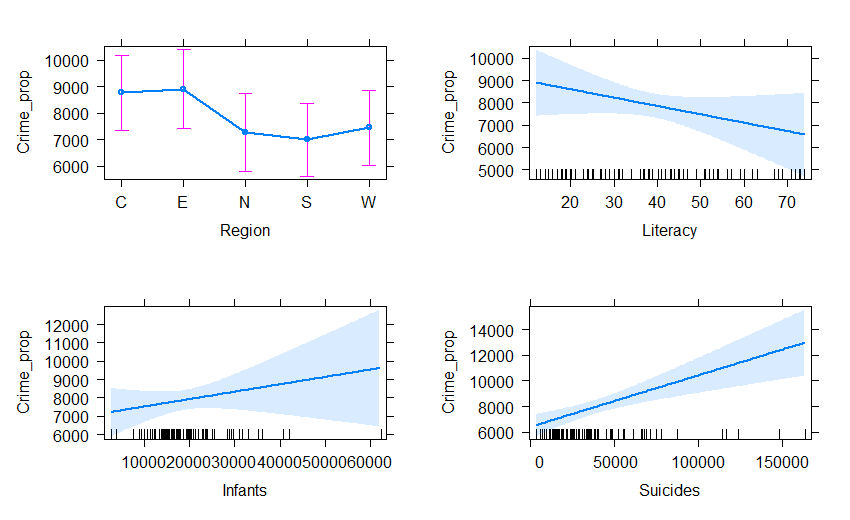## Predicting crime: Multivariate regression The two regression models can be fit together in a multivariate regression for both crime variables jointly. crime.mod <- lm(cbind(Crime_pers, Crime_prop) ~ Region + Literacy + Donations + Infants + Suicides, data=Guerry) Anova(crime.mod) #> #> Type II MANOVA Tests: Pillai test statistic #> Df test stat approx F num Df den Df Pr(>F) #> Region 4 0.42933 5.1936 8 152 9.563e-06 *** #> Literacy 1 0.03707 1.4434 2 75 0.2425951 #> Donations 1 0.02615 1.0071 2 75 0.3701736 #> Infants 1 0.01833 0.7001 2 75 0.4997450 #> Suicides 1 0.20772 9.8315 2 75 0.0001615 *** #> --- #> Signif. codes: 0 '***' 0.001 '**' 0.01 '*' 0.05 '.' 0.1 ' ' 1 As a quick check on the assumption that the residuals are bivariate normally distributed and a check for outliers, a $$\chi^2$$ Q-Q plot graphs the squared Mahalanobis distances of the residuals against the corresponding $$\chi^2_2$$ quantiles these would have in a bivariate normal distribution. The data for Creuse stands out as a potential outlier. labels <- paste0(Guerrydept,":", Guerry\$Department)
cqplot(crime.mod, id.n=4, labels=labels)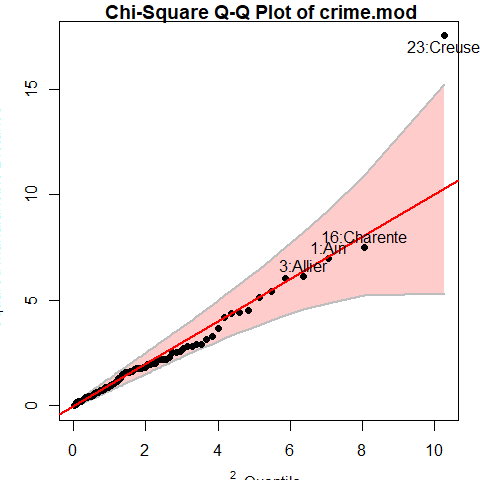### HE plots

Hypothesis-Error (HE) plots provide a convenient graphical summary of hypothesis tests in multivariate linear model. They plot a data ellipse for the residuals in the model, representing the $$\mathbf{E}$$ matrix in the test statistics (Roy’s maximum root test, Pillai and Hotelling trace criteria and Wilks’ Lambda). Overlaid on this are $$\mathbf{H}$$ ellipses for each term in the model, representing the data ellipses for the fitted values. Using Roy’s test, these have a convenient interpretation: a term is significant iff the H ellipse projects anywhere outside the E ellipse. For a 1 df (regression) variable, the H ellipse collapses to a line.

heplot(crime.mod,
fill=TRUE, fill.alpha=0.05,
cex=1.4, cex.lab=1.3 )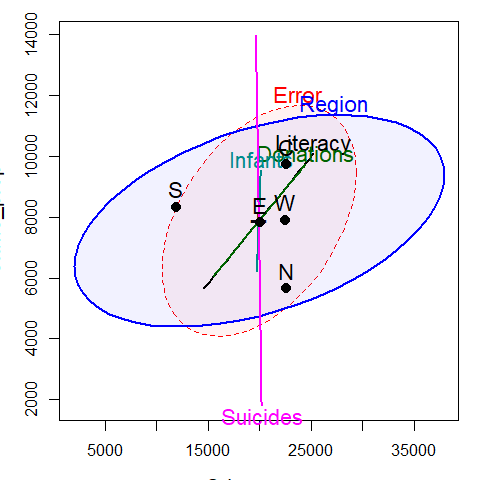In this plot, the effect of Suicides is completely aligned with crimes against property. The effect of Region is positively correlated with both types of crime. The means for the regions show that the South of France is lower (worse) on personal crime; the other regions vary most in property crime, with the North being lower and the Center being higher.

### Canonical plots

The HE plot displays these relations in data space. An alternative is provided by canonical discriminant analysis, which finds the weighted sums of the response variables leading to the largest test statistics for the terms, which can be visualized in canonical space.

The analysis below reflects the effect of Region in relation to both crime variables.

crime.can <- candisc(crime.mod)
crime.can
#>
#> Canonical Discriminant Analysis for Region:
#>
#>     CanRsq Eigenvalue Difference Percent Cumulative
#> 1 0.337068    0.50845    0.40681   83.34      83.34
#> 2 0.092267    0.10164    0.40681   16.66     100.00
#>
#> Test of H0: The canonical correlations in the
#> current row and all that follow are zero
#>
#>   LR test stat approx F numDF denDF   Pr(> F)
#> 1      0.60177   5.7097     8   158 2.209e-06 ***
#> 2      0.90773   2.7105     3    80   0.05051 .
#> ---
#> Signif. codes:  0 '***' 0.001 '**' 0.01 '*' 0.05 '.' 0.1 ' ' 1

The HE plot for this analysis is shown below. Variable vector represent the correlations of the crime variables with the canonical dimension.

heplot(crime.can, fill=TRUE, fill.alpha=0.1,
var.col = "black",
var.cex = 1.3,
cex=1.4, cex.lab=1.3)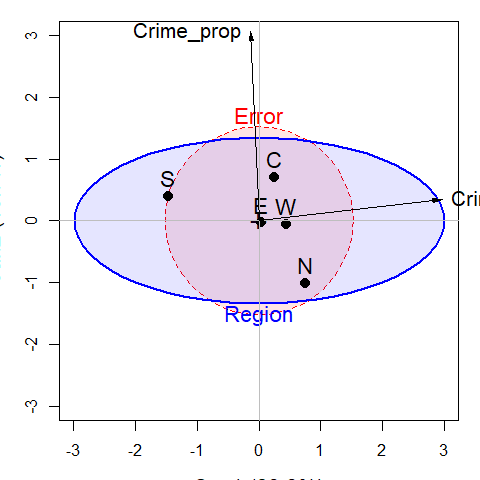#> Vector scale factor set to  3.10537

This gives a simple interpretation of the differences in Region on the crime variables. The first canonical dimension accounts for 83% of differences among the regions, and this is nearly perfectly aligned with personal crime, with the largest difference between the South and the other regions. The second canonical dimension, accounting for the remaing 17%, is perfectly aligned with property crime. On this dimension, the North stands out compared to the other regions.